# Test: MCQs (One or More Correct Option): Miscellaneous (Sets, Relations, Statistics & Mathematical Reasoning) | JEE Advanced

## 2 Questions MCQ Test Maths 35 Years JEE Main & Advanced Past year Papers | Test: MCQs (One or More Correct Option): Miscellaneous (Sets, Relations, Statistics & Mathematical Reasoning) | JEE Advanced

Description
Attempt Test: MCQs (One or More Correct Option): Miscellaneous (Sets, Relations, Statistics & Mathematical Reasoning) | JEE Advanced | 2 questions in 5 minutes | Mock test for JEE preparation | Free important questions MCQ to study Maths 35 Years JEE Main & Advanced Past year Papers for JEE Exam | Download free PDF with solutions
*Multiple options can be correct
QUESTION: 1

### In a college of 300 studen ts ever y student r eads 5 newspapers and every newspaper is read by 60 students.The number of newpapers is (1998 - 2 Marks)

Solution:

(c) Let n be the number of newspapers which are read.
Then 60 n = (300) (5) ⇒ n = 25

*Multiple options can be correct
QUESTION: 2

### Let S1, S2, ...... be squares such that for each n ≥ 1, the length of a side of Sn equals the length of a diagonal of Sn+1. If the length of a side of S1 is 10 cm, then for which of the following values of n is the area of Sn less than 1 sq. cm? (1999 - 3 Marks)

Solution:

Given side of square S1 = 10 cm As it is same as diagonal of S2.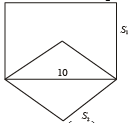∴ Side of square S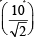Similarly, side of square S3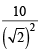In the same manner, side of square Sn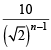∴ Area of square of side Sn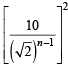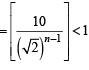⇒ 100 < (2) n–1 ⇒ 2n–1 > 100 ⇒ n – 1 ∈ 7 ⇒  n ∈ 8Use Code STAYHOME200 and get INR 200 additional OFF Use Coupon Code